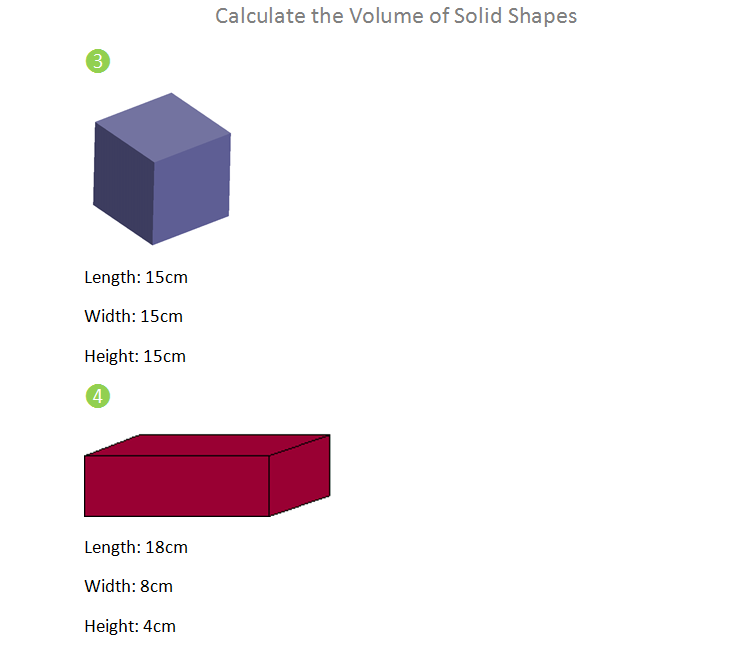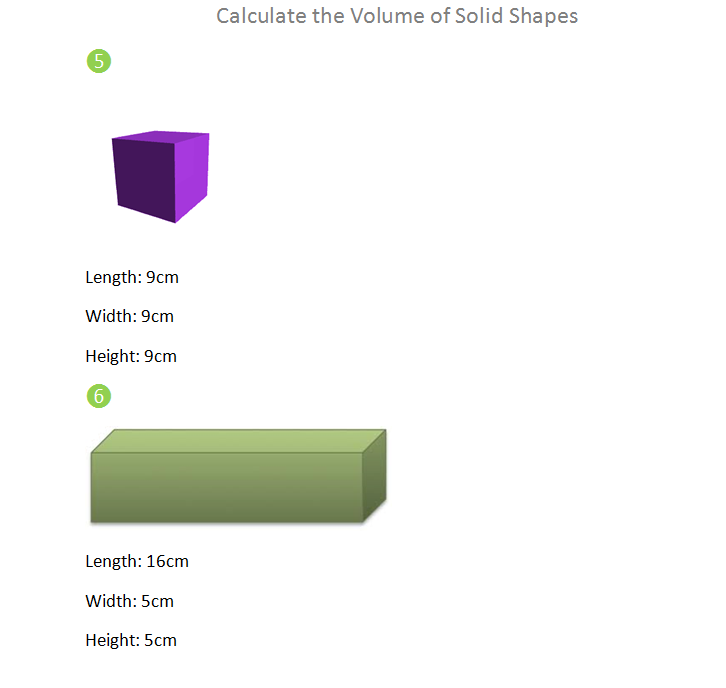## Preview

Calculating the volume of solid shapes is just as easy as finding the area of plane shapes because all that applies in the calculation of volume is width x length x height.

However you could say that volume is also a unit of math in which measurement is required.
So for example if have the cube to find the volume of and the measurements are already given which are:

4 inches x 12 inches x 3 inches

The only step in calculating the volume is multiplying the measurements.
So, 4x12x3 is equals to 144.
Our volume is 144 inches cubed.

Sheet 1 How to Calculate the Volume of Solid ShapesSheet 2 Practice Problems on Calculating the Volume of Solid ShapesSheet 3 Volume of Solid Shapes